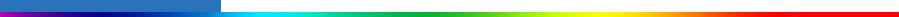Working Group I: The Scientific BasisGet Javascript Other reports in this collection

#### 6.15.2.4 Aerosol indirect effect

The indirect radiative forcing was calculated by the LLNL/Umich model (Chuang et al., 2000a), the GISS model (Koch et al., 1999), and the Max Planck/Dalhousie (Lohmann et al., 1999a,b, 2000) model. The LLNL/Umich model uses the mechanistic formulation for the determination of droplet concentration described by Chuang et al. (1997) but has been updated to include interactive dust and sea salt. This model provides an estimate of the “first” indirect effect. The GISS model used an empirical formulation for relating droplet concentrations and aerosol concentrations and also provides an estimate of the “first” indirect effect. The Max-Planck/Dalhousie model used the mechanistic formulation of Chuang et al. (1997) as described in Lohmann et al. (1999a,b, 2000) and includes both the “first” and “second” indirect effects. In addition, the Max-Planck/Dalhousie model used monthly averaged dust and sea salt fields from the LLNL/Umich model. Further details of the models are provided in Chapter 5. The indirect radiative forcing scenarios are summarised in Table 6.16, and represent the radiative forcing from the year 2000 due to sulphate and carbonaceous aerosols. Some simulations suggest that changes in the concentrations of natural aerosols due to changes in the climate could also contribute to a negative aerosol indirect effect (see Chapter 5).

As for the aerosol direct effect, the uncertainty associated with these estimates is higher than for the estimates for the present day. Considering the very low LOSU of the indirect aerosol effect and the fact that no best estimate was recommended for the present day (Sections 6.8 and 6.13), the numbers provided in Table 6.16 give only a rough indication of how the forcings could change between the present and the future (according to the SRES scenarios) in three different models. Relative to 2000, the change in the direct plus indirect aerosol radiative forcing is projected to be smaller in magnitude than that of CO2.

 Table 6.16: Indirect aerosol radiative forcing (Wm-2) estimated by different models for the IPCC SRES scenarios described in Chapter 5. The sulphate burdens in each case are given in Chapter 5, Table 5.14. These estimates are from the average difference in cloud forcing between two simulations. The numbers represent the radiative forcing from 2000. No numbers are given for the year 2000 as no best estimate of the radiative forcing is suggested in Section 6.8.LLNL/Umich GISS Max Planck/DalhousieA2 2030 -0.24 -0.29 -0.05 A2 2100 -0.47 -0.36 -0.32 B1 2100 +0.10